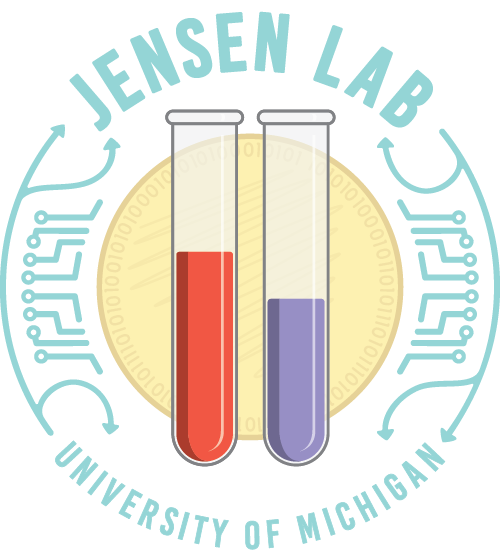## Books

#### Linear Algebra: Foundations of Machine Learning

This book offers a gentle introduction to the algebra of vectors and high-dimensional systems. Selected topics from linear algebra, optimization, and nonlinear systems prepare readers for advanced coursework in machine learning and data science.

The book grew out of a sophomore-level courses on biomedical data science at the University of Illinois (BIOE 210) and the University of Michigan. A free PDF of the Fall 2022 edition is available here. Instructor resources are available upon request.

• Part I: Linear Systems
• Fields and vectors
• Matrices
• Rotation and translation matrices
• Solving linear systems
• The Finite Difference Method
• Matrix inverses
• Rank and solvability
• Linear models and regression
• Building regression models
• Part II: Nonlinear Systems
• Root finding
• Optimization and convexity
• Logistic regression
• Bias, variance, and regularization
• Geometry
• Support Vector Machines
• Part III: High-Dimensional Systems
• Vector spaces, span, and basis
• Eigenvectors and eigenvalues
• Matrix decompositions
• Low-rank approximations

## Courses

#### BIOE 210: Linear Algebra for Biomedical Data Science

Using analytical and computational tools from linear algebra, students in this course will

• Solve large systems of linear equations, systems of linear ODEs, and linear PDEs.
• Analyze large, multivariable datasets to quantify relationships between variables.
• Decompose complex datasets into simpler representations.
• Introduce and solve common problems in classification, image processing, and machine learning.
• Develop a geometric understanding of high-dimensional spaces.

The course website for Spring 2022 is available here.

#### BIOE 498/598 PJ: Experiment Design & Optimization

All engineers perform experiments. Whether they be “wet-lab” or simulated, experiments test the limits of our hypotheses and drive our understanding. Often engineers want to go beyond validating their theories and models. Engineers want the best designs – the optimal combination of cost, reliability, performance, and usability.

Part I of this course presents Design of Experiments and Response Surface Methodology, two systematic frameworks for optimizing and understanding real-world systems. Using both experimental data and computer simulations, students will explore methods to efficiently search large design spaces. Upon completion, students will be able to

• Design experiments with maximum statistical power.
• Use sequential experiments to find optimal experimental conditions.
• Tune “black box” models with multiple inputs and parameters.

Part II generalizes the above techniques into Surrogate Optimization to tackle larger problems with complex response surfaces. Surrogate optimization emphasizes active learning and sequential experiments that adapt designs based on intermediate results.

Part III introduces Reinforcement Learning, a branch of artificial intelligence where agents both design experiment and interpret the results. Reinforcement learning underlies many recent AI breakthroughs in gameplay, logistics, protein folding, self-driving cars, and robotics. Students will learn to build agents that rival human experts when solving multistage decision problems.

The techniques in this course apply to any field of science and engineering. The course will focus on biomedical and bioengineering topics, including

• Bioprocess and metabolic engineering
• Medical device design and testing
• Optimizing molecular biology assays
• Design of synthetic gene circuits
Students will use real-world data and simulation software to optimize designs. As is typical in engineering, many of the projects lack a single “best” answer. Students will learn to balance multiple objectives and constraints when solving problems.

The course website for Spring 2022 is available here.